﻿ 基于<i>K</i>均值聚类EEMD的CORS高程时间序列信号分析方法文章快速检索 高级检索
 大地测量与地球动力学2019, Vol. 39Issue (10): 1053-1057  DOI: 10.14075/j.jgg.2019.10.013### 引用本文ZHANG Hengjing, QI Xin, WEN Hanjiang. Analytical Method of CORS Height Time Series Based on K-Means Clustering EEMD[J]. Journal of Geodesy and Geodynamics, 2019, 39(10): 1053-1057.### Foundation support

The Major Project of High Resolution Earth Observation System, No.42-Y20A09-9001-17/18; Fundamental Research Funds for Universities of Education Department of Liaoning Province, No.LJ2017QL008; PhD Start-Up Fund of Liaoning Technical University, No.13-1120.

### Corresponding author

QI Xin, postgraduate, majors in spatial geodetic data processing, E-mail: lntuqixin@163.com.

### 第一作者简介

ZHANG Hengjing, PhD, associate professor, majors in spatial geodetic data processing and nonlinear motion of GNSS height time series, E-mail:sun_winter2009@163.com.

### 文章历史

1. 辽宁工程技术大学测绘与地理科学学院，阜新市玉龙路88号，123000;
2. 中国测绘科学研究院，北京市莲花池西路28号，100830

1 改进的EEMD算法 1.1 基于正负白噪声的EEMD算法 1.1.1 EEMD算法

EEMD的基本思路是在分解前将原始序列信号加入高斯白噪声生成新的待分解信号，对多个本征模态函数(IMF)分量进行平均处理，进而保留具有物理意义的IMF分量，同时消除加入的噪声。对CORS高程序列添加白噪声：

 ${X_i}\left( t \right) = X\left( t \right) + {w_i}\left( t \right)$ (1)

 ${n_{{\rm{max}}}} = {\rm{fix}}({\rm{lo}}{{\rm{g}}_2}N) - 2$ (2)

1.1.2 添加正负白噪声

 ${X_i}\left( t \right) = X\left( t \right) + {\left( { - 1} \right)^q}{d_0}{w_i}\left( t \right)$ (3)

1.2 K均值聚类算法

K均值聚类分析的EEMD算法采用划分聚类方法中的K均值算法，将每次EEMD循环产生的IMF分量进行聚类分析，保留聚类个数多的一类，取该类中IMF分量的平均值作为分解产生的IMF。K均值聚类算法首先要确定常数K为最终分类的数量。令K从2到某个固定值，在每个K值上运行数次K聚类，避免局部最优解，计算K的轮廓系数，最后选取轮廓系数最大的值对应的K作为最终分类个数。轮廓系数计算方法为：

 $S\left( \mathit{\boldsymbol{i}} \right) = \frac{{o\left( \mathit{\boldsymbol{i}} \right) - p\left( \mathit{\boldsymbol{i}} \right)}}{{{\rm{max}}\left[ {p\left( \mathit{\boldsymbol{i}} \right), o\left( \mathit{\boldsymbol{i}} \right)} \right]}}$ (4)

 $E({a_1}, \ldots , {a_k}) = \frac{1}{n}\sum\limits_{i = 1}^k {\sum\limits_{m \in {a_i}} {{{\left\| {{m_j} - {a_i}} \right\|}^2}} }$ (5)

 $\begin{array}{*{20}{c}} {{D_{(A, B)}} = }\\ {\sqrt {{{({A_1} - {B_1})}^2} + {{({A_2} - {B_2})}^2} + \ldots + {{({A_n} - {B_n})}^2}} } \end{array}$ (6)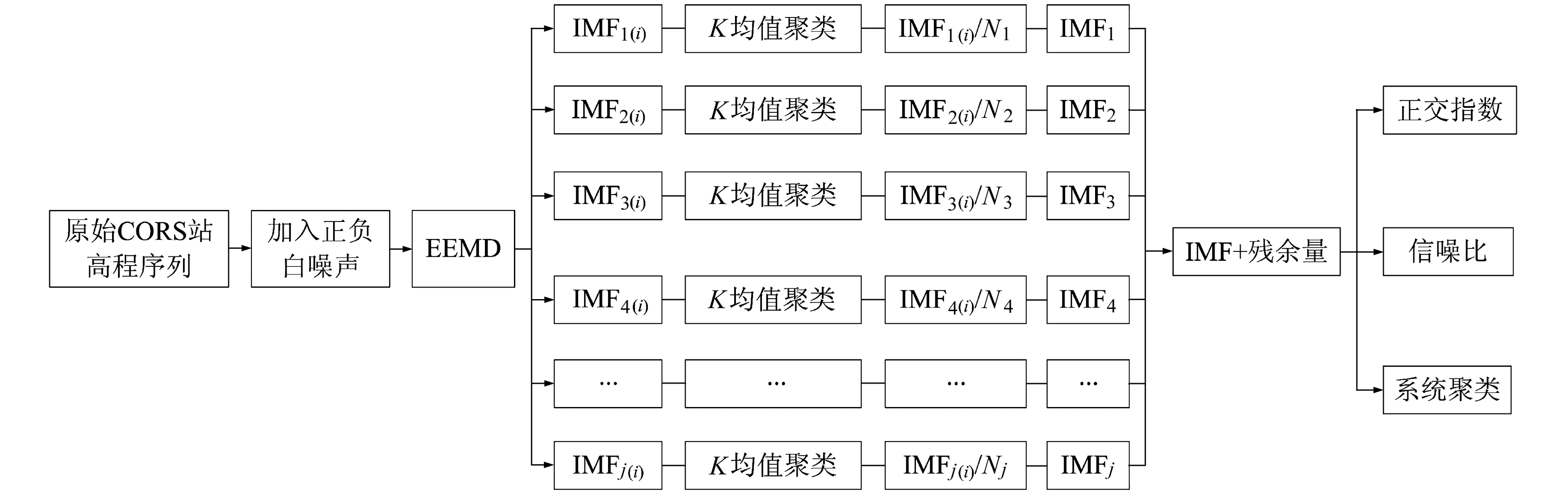图 1 K均值聚类EEMD流程 Fig. 1 K-means clustering EEMD flowchart
1.3 正交指数与信噪比指标

 ${{\rm{IO}}_{ij}} = \sum\limits_t {\frac{{{C_i}\left( t \right){C_j}\left( t \right)}}{{{C}_i^2\left( t \right) + {C}_j^2\left( t \right)}}}$ (7)

CORS高程时间序列信号的信噪比(SNR)计算的是EEMD加入白噪声分解前后的去噪效果，计算公式为：

 ${\rm{SNR}} = 10{\rm{lo}}{{\rm{g}}_{10}}\left( {\frac{{E\left[ {y{{\left( n \right)}^2}} \right]}}{{E\{ {{\left[ {y\left( n \right) - \hat y\left( n \right)} \right]}^2}\} }}} \right)$ (8)

1.4 方差贡献率

 $\rho _x^2 = E({x^2}) - {E^2}\left( x \right)$ (9)
2 实验分析 2.1 EEMD和K均值EEMD分解实验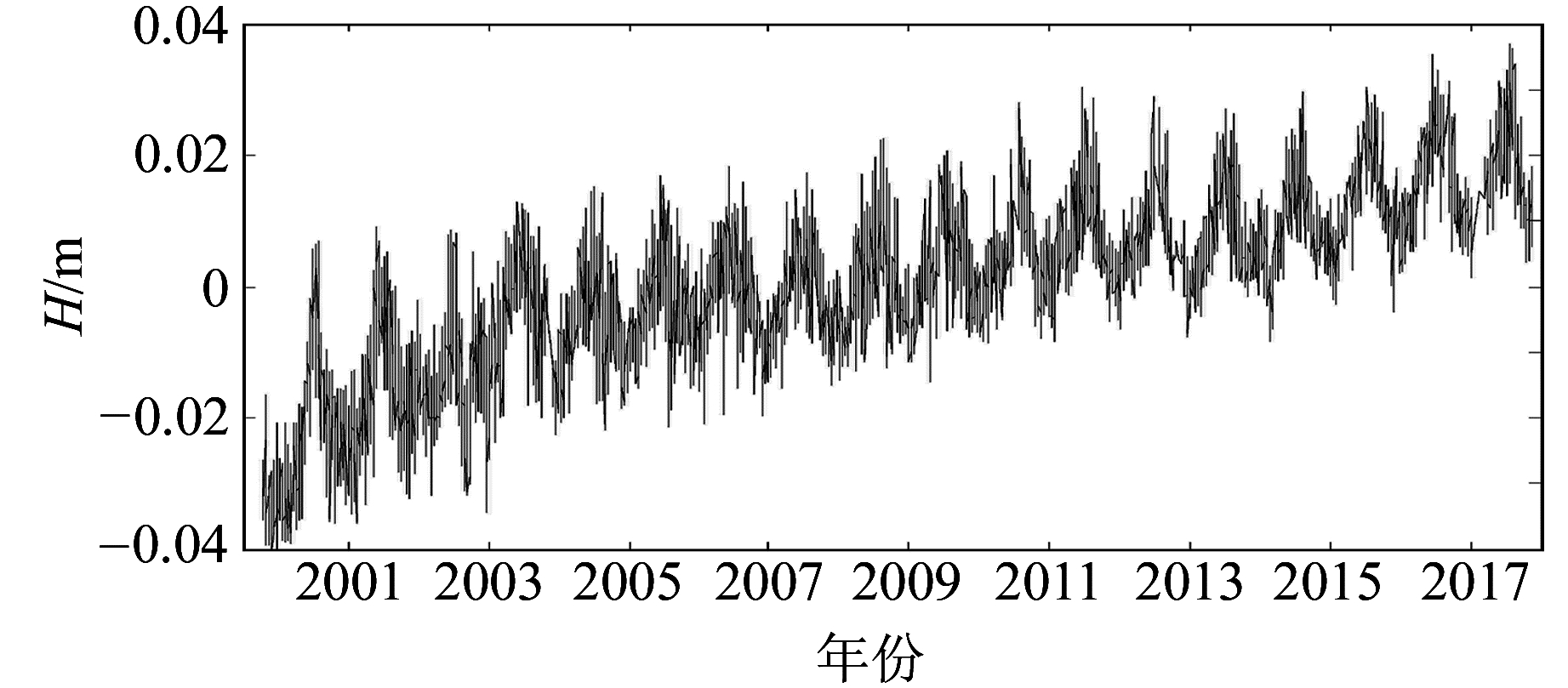图 2 BJFS站原始高程序列 Fig. 2 Height time series of BJFS station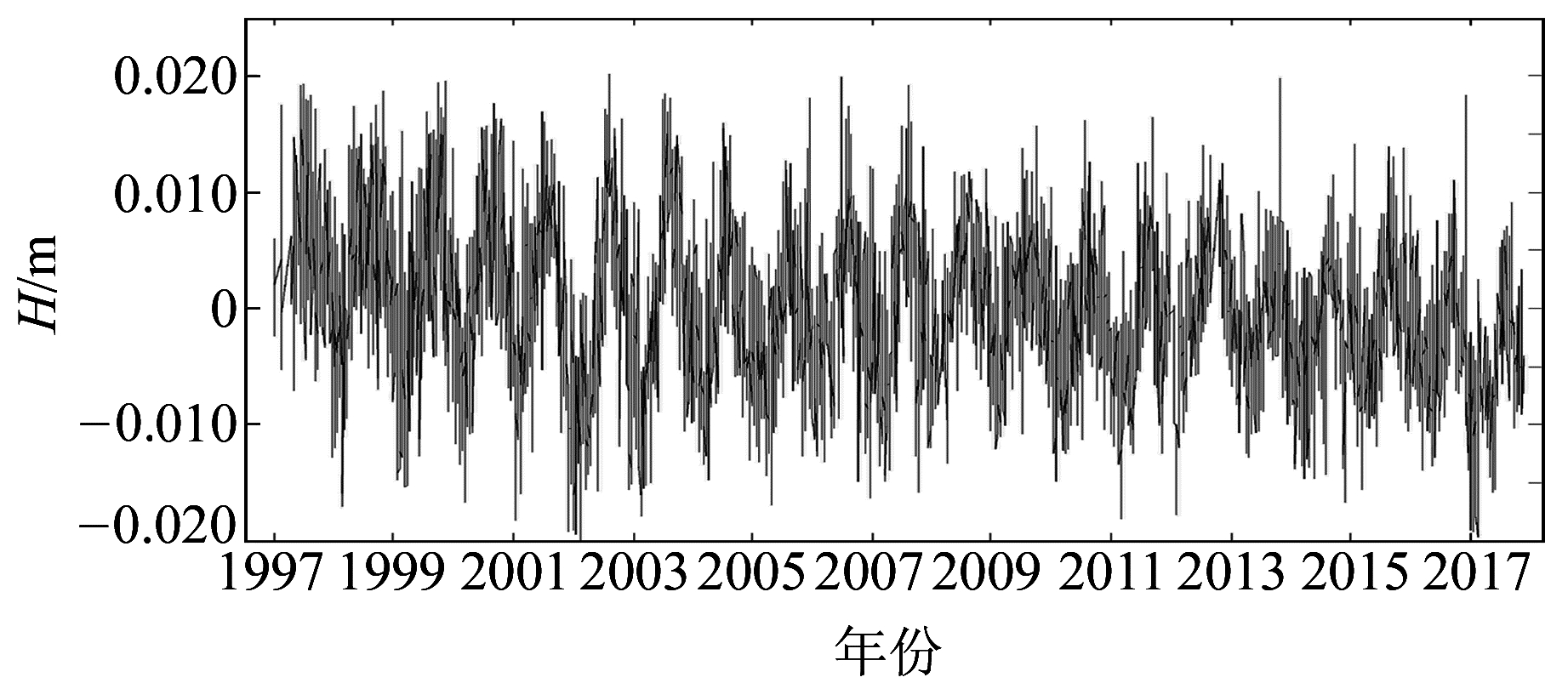图 3 BOGO站原始高程序列 Fig. 3 Height time series of BOGO station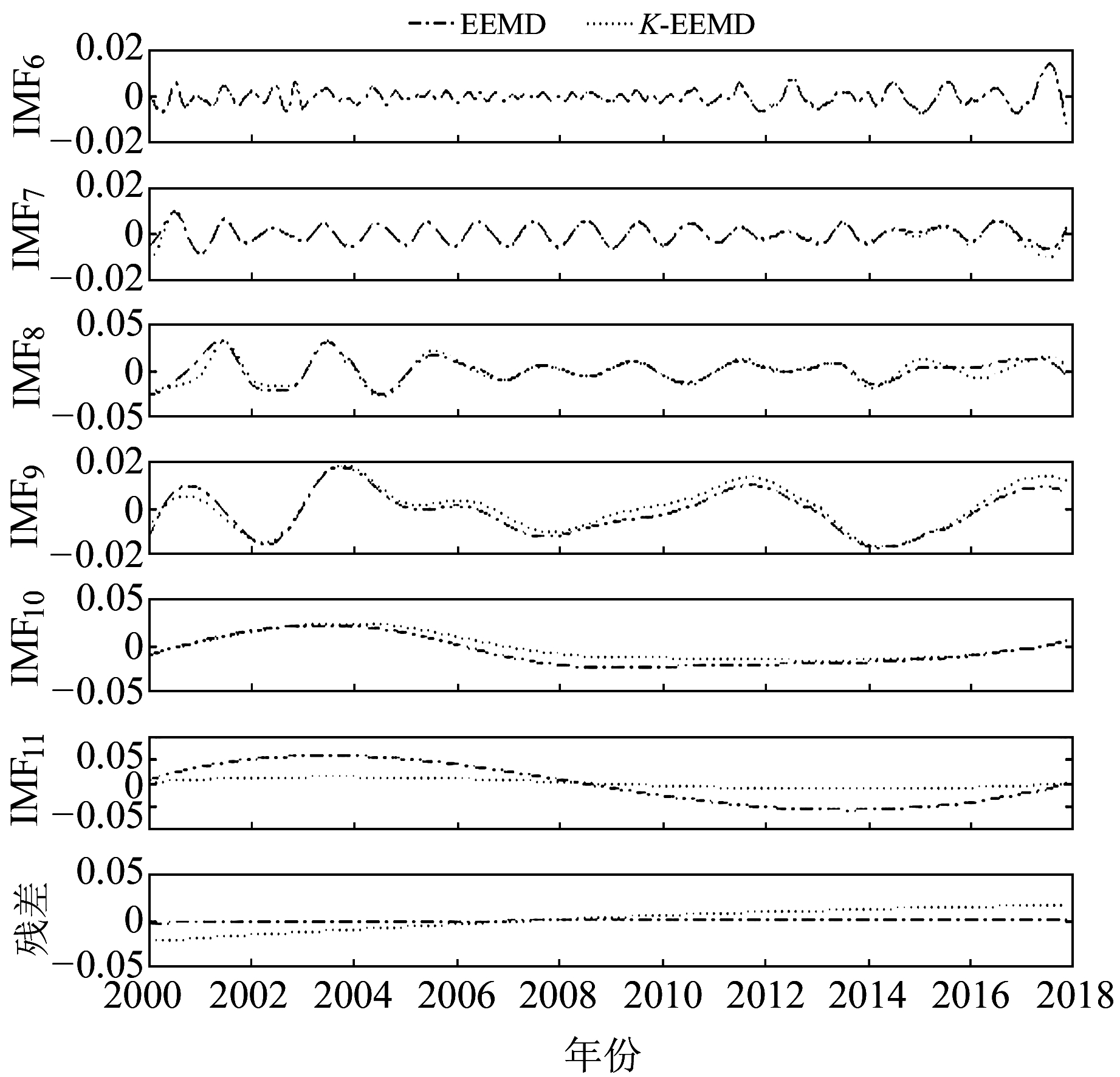图 4 BJFS站IMF6~IMF11分量 Fig. 4 IMF6-IMF11 components of BJFS station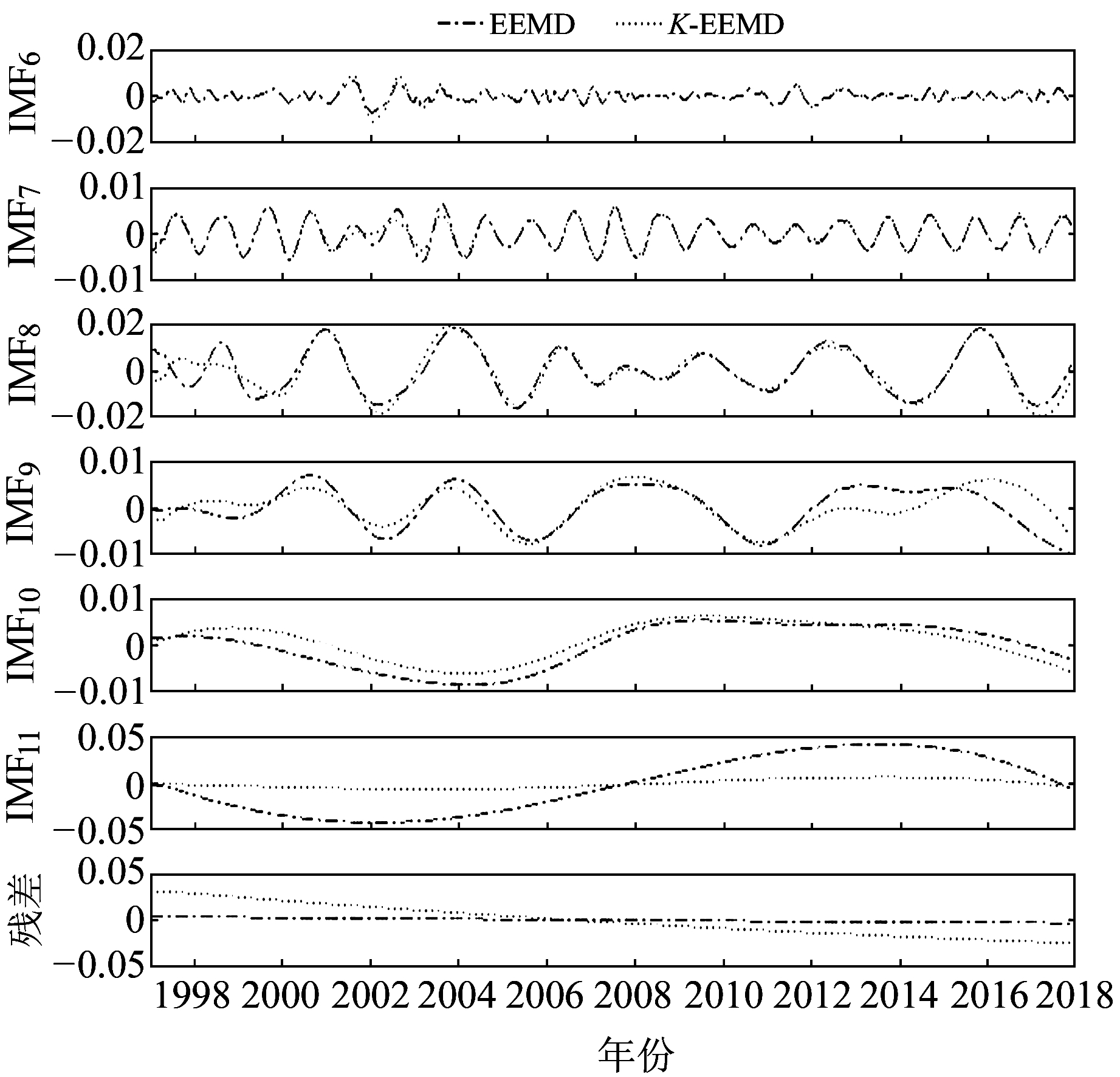图 5 BOGO站IMF6~IMF11分量 Fig. 5 IMF6-IMF11 components of BOGO station表 1 2个测站各IMF分量的方差贡献率 Tab. 1 The variance contribution rate of each IMF

BJFS站IMF1~IMF3为明显高频分量，主要是信号中的有色噪声；IMF4~IMF5的方差贡献率分别为5.43%和3.59%，在序列中占比较小；IMF6与IMF7的方差贡献率分别为21.19%、30.59%，在序列中占比最大，为序列主要周期项。从图 4可以看出，IMF6为近似0.5 a周期项，IMF7为近似1 a周期项，IMF8为近似2 a周期项，IMF9~IMF11周期明显变长，趋势项单调性明显，符合经验模式分解的要求。

BOGO站分解后与BJFS站类似，IMF6与IMF7的方差贡献率分别为20.12%、22.86%，分别为近似0.5 a和1 a的主要周期贡献项；IMF8方差贡献率2.85%，为近似2 a周期项。除IMF1~IMF3为高频分量外，其余各分量方差贡献率大致相同。

2.2 精度对比表 2 2种分解方法的正交指数与信噪比 Tab. 2 IO67 and SNR of EEMD and K-EEMD

2.3 IMF系统聚类分析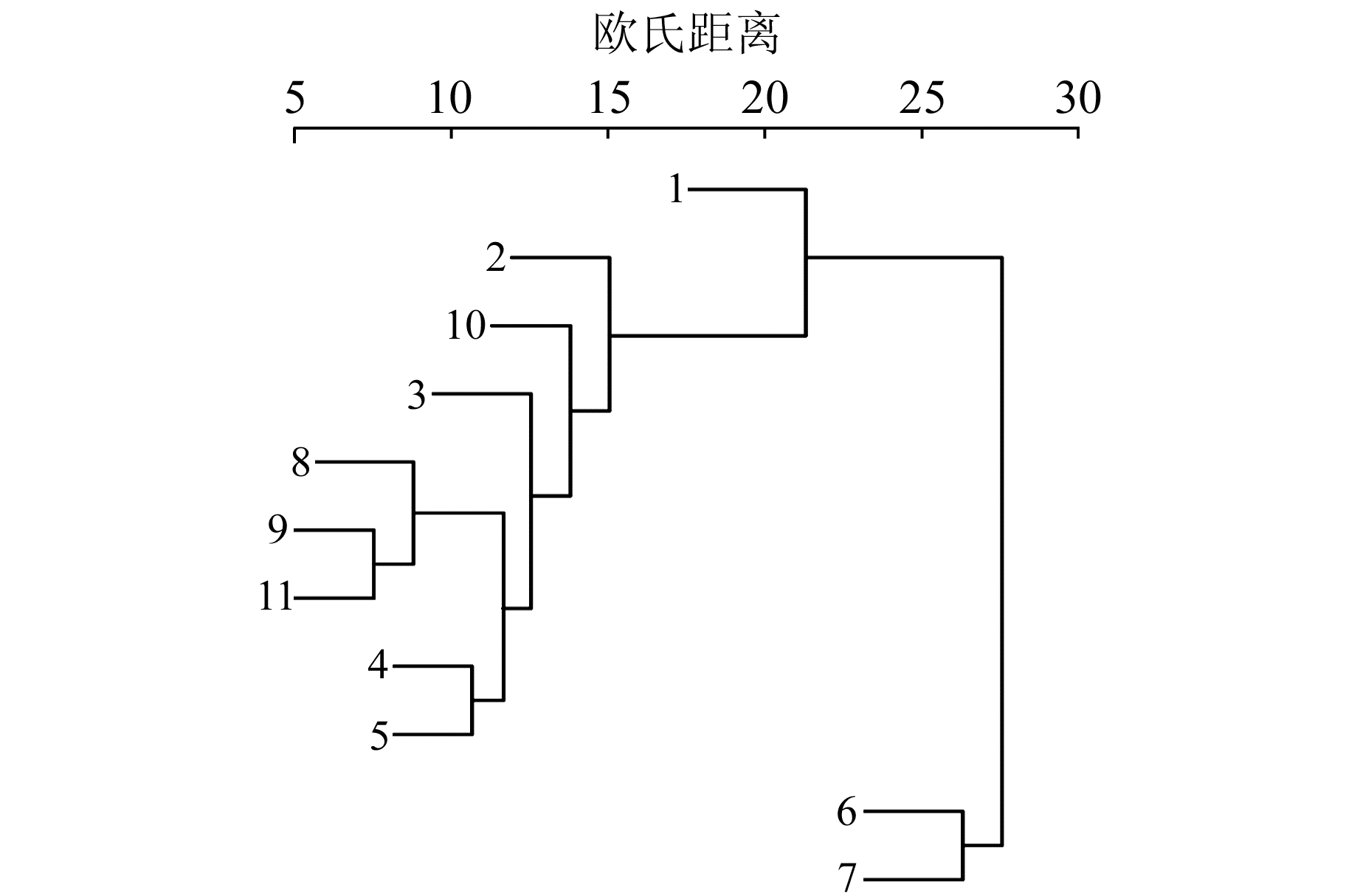图 6 EEMD方法BJFS站IMF聚类图 Fig. 6 IMF clustering by EEMD of BJFS station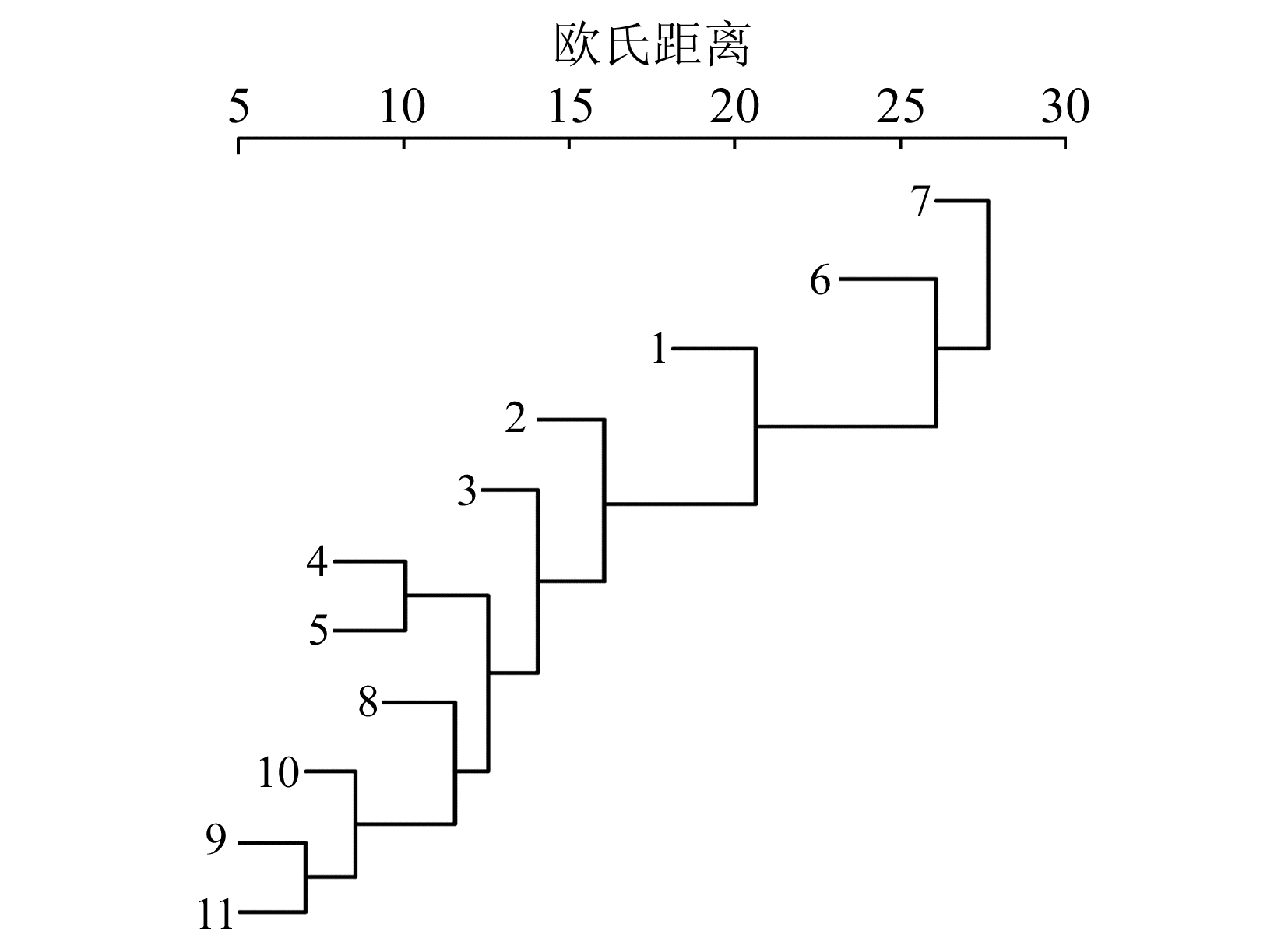图 7 K-EEMD方法BJFS站IMF分量聚类图 Fig. 7 IMF clustering by K-EEMD of BJFS station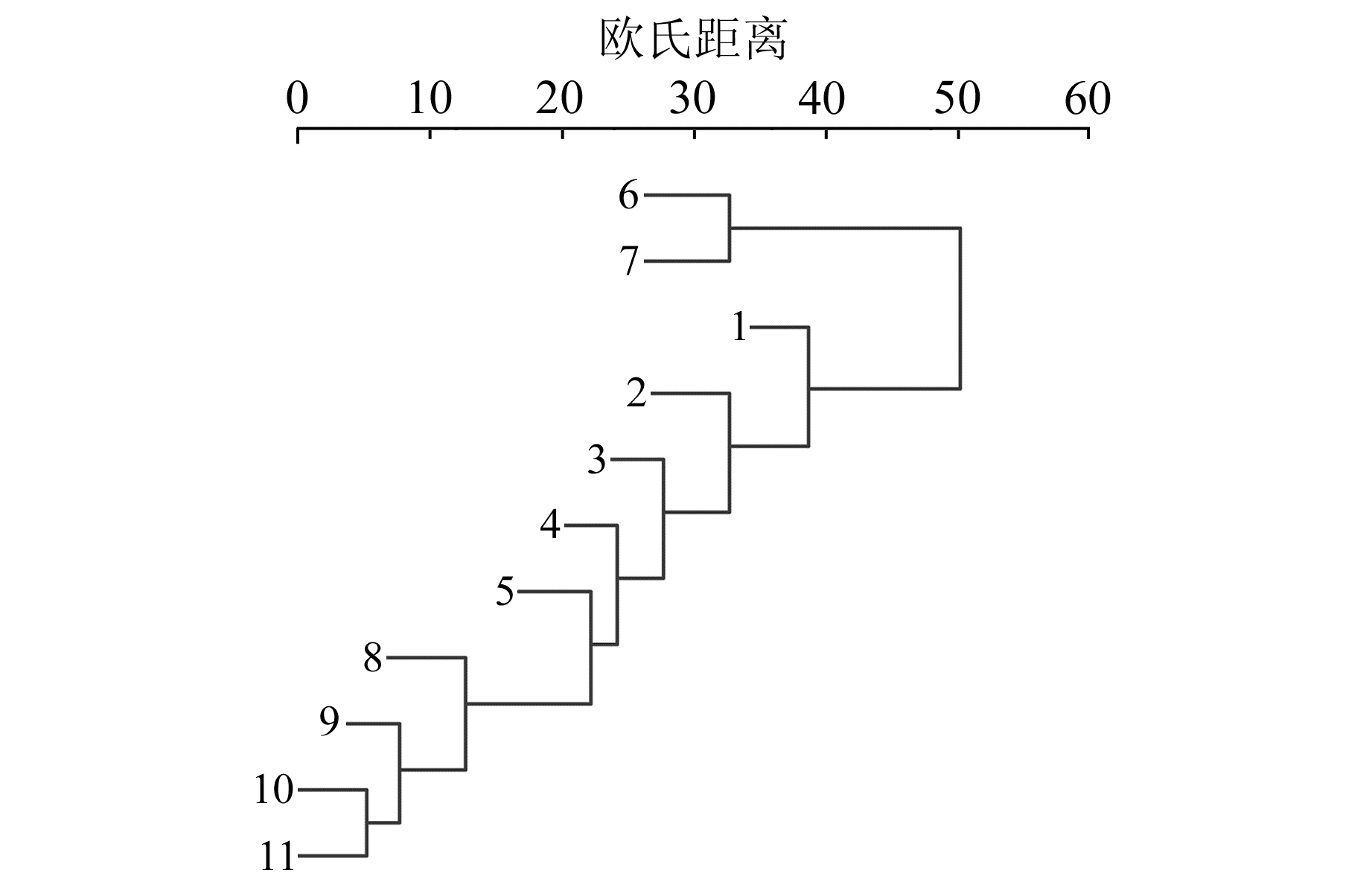图 8 EEMD方法BOGO站IMF聚类图 Fig. 8 IMF clustering by EEMD of BOGO station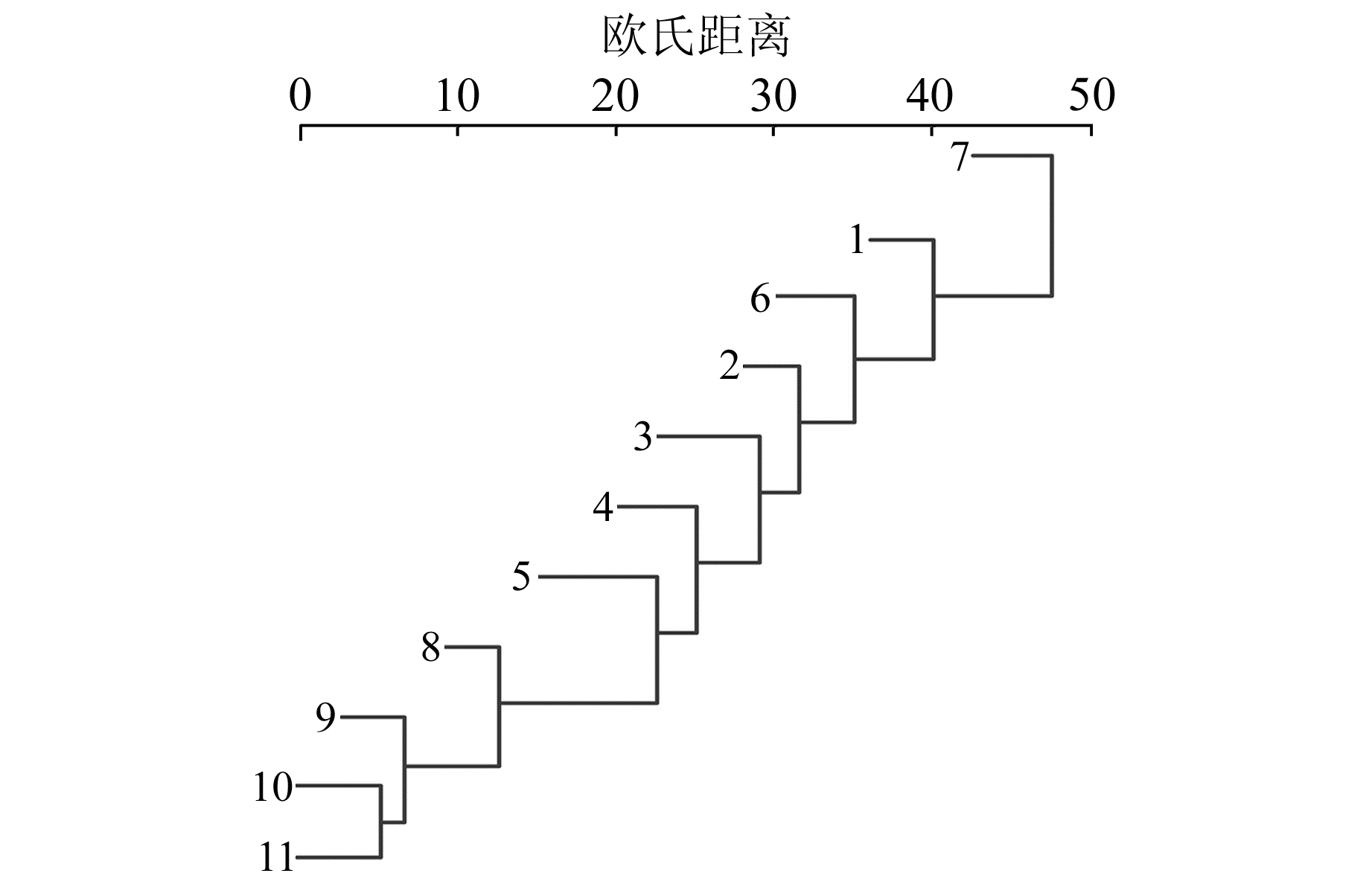图 9 K-EEMD方法BOGO站IMF聚类图 Fig. 9 IMF clustering by K-EEMD of BOGO station

2个CORS站的实验结果表明，EEMD分解后IMF6与IMF7之间存在模态混叠现象，而K均值聚类EEMD在同等条件下不存在模态混叠现象。CORS高程时间序列的IMF分量包含高频噪声项、周期项和低频趋势项。中低频IMF分量对高程时间序列信号的周期运动给出清晰的解释，包含明显的季节性、1 a和2 a周期变化、长周期变化。已有研究表明，IMF周期信号通过Hilbert变换后的频率并不是一个常数，而是瞬时频率，说明IMF的周期随时间而变化，这个问题需要进一步研究。

3 结语

  Wu Z H, Huang N E. Ensemble Empirical Mode Decomposition: A Noise-Assisted Data Analysis Method[J]. Advances in Adaptive Data Analysis, 2009, 1(1): 1-41 (0)  徐健, 周志祥, 唐亮, 等. 基于总体平均经验模态分解算法的自适应改进[J]. 振动与冲击, 2017, 36(11): 215-223 (Xu Jian, Zhou Zhixiang, Tang Liang, et al. Adaptive Improvement of EEMD Algorithm[J]. Journal of Vibration and Shock, 2017, 36(11): 215-223) (0)  郑近德, 程军圣, 杨宇. 改进的EEMD算法及其应用研究[J]. 振动与冲击, 2013, 32(21): 21-26 (Zheng Jinde, Cheng Junsheng, Yang Yu. Modified EEMD Algorithm and Its Applications[J]. Journal of Vibration and Shock, 2013, 32(21): 21-26 DOI:10.3969/j.issn.1000-3835.2013.21.004) (0)  施闯, 牛玉娇, 魏娜, 等. HHT-EEMD用于IGS站高程时间序列分析[J]. 大地测量与地球动力学, 2018, 38(7): 661-667 (Shi Chuang, Niu Yujiao, Wei Na, et al. Application of the HHT-EEMD Approach in Analysis of GPS Height Time Series[J]. Journal of Geodesy and Geodynamics, 2018, 38(7): 661-667) (0)  李鹏.基于层次K均值的聚类算法的研究[D].哈尔滨: 哈尔滨工程大学, 2015 (Li Peng. The Study and Development of Hierarchical K-Means Based Clustering Algorithm[D]. Harbin: Harbin Engineering University, 2015) (0)  Chung K L, Lin J S. Faster and More Robust Point Summetry-Based K-Means Algorithm[J]. Pattern Recognition, 2007, 40(2): 410-422 DOI:10.1016/j.patcog.2005.09.015 (0)  Rousseeuw P J. Silhouettes: A graphical Aid to the Interpretation and Validation of Cluster Analysis[J]. Journal of Computational and Applied Mathematics, 1987, 20: 53-65 DOI:10.1016/0377-0427(87)90125-7 (0)  唐东明.聚类分析及其应用研究[D].成都: 电子科技大学, 2010 (Tang Dongming. Study on Clustering Algorithm and Its Applications[D]. Chengdu: University of Electronic Science and Technology, 2010) http://cdmd.cnki.com.cn/Article/CDMD-10614-2010234406.htm (0)  Huang N E, Shen Z, Long S R, et al. The Empirical Mode Decomposition and the Hilbert Spectrum for Nonlinear and Non-Stationary Time Series Analysis[J]. The Royal Society, 1998, 454(1 971): 903-995 (0)  张恒璟, 程鹏飞. 基于经验模式分解的CORS站高程时间序列分析[J]. 大地测量与地球动力学, 2012, 32(3): 129-134 (Zhang Hengjing, Cheng Pengfei. Analysis on Time Series of Two CORS Stations' Height Based on EMD[J]. Journal of Geodesy and Geodynamics, 2012, 32(3): 129-134) (0)
Analytical Method of CORS Height Time Series Based on K-Means Clustering EEMD
ZHANG Hengjing1,2QI Xin1,2WEN Hanjiang2
1. School of Geomatics, Liaoning Technical University, 88 Yulong Road, Fuxin 123000, China;
2. Chinese Academy of Surveying and Mapping, 28 West-Lianhuachi Road, Beijing 100830, China
Abstract: We advance an analytical method of CORS height time series based on K-means clustering EEMD. Aiming at the problem of low signal-to-noise ratio and partial mode mixing in signal decomposition of traditional EEMD, we add positive and negative white noise EEMD to improve signal decomposition SNR. Based on the K-means clustering method, we cluster the various IMF components decomposed in the EEMD iterative process. The experience results show that the method improves the SNR by more than 3%, and the decomposition accuracy based on index of orthogonality increases by more than 26%. The clustering results can solve the problem of mode mixing of the approximate year, half-year and two years periodic signals in the IMF.
Key words: height time series; mode mixing; K-means clustering; SNR; index of orthogonality